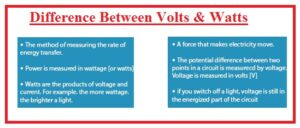Hello, friends, I hope you all are doing great. In today’s tutorial, we will discuss the Difference Between Volts & Watts. The basic difference between volts and watts is that system international unit of potential difference end electromotive force is volts and the unit of power is the watt. Either both are different units but have some relation with each other. The volts calculate the value of the voltage of the electric instrument and it denoted as V.

The calculations find through the watt is difficult than the volts since watt is multiple of two quantities voltage and current. Watt is denoted with the W. It used to find the value of power consumed by the instrument. In today’s post, we will have a detailed look at volts and watts and compare them to find their differences. So let’s get started with Difference Between Volts & Watts.

#### Difference Between Volts & Watts

Volts

• The SI unit of potential difference and volts is known as volts.
• Its formulas is V= electrical energy/ charge
• Voltage is the energy required by the charge to move from one location to another.
• Its formula is V=IR. and define J/C here J is joule and C is coloumb
• Its symbolic representation is V. Its other units are millivolts, kilovolts,
• Voltmeter used to finds its value
• Volts help to find electromotive force and potential difference
• Volts is generating through the electrical field
• The value of voltage can be found between two points.
• The base unit of a volt is KgM2S-3
• This unit calculates the small quantity of voltage from the power source.

Watt

• It is unit used to meaure power alos units of radiantflux.
• Its units is 1 kg⋅m2⋅s−3
• That is eqal to one J/S
• This term helps to finds the value of energy tranfer during circuitry.
• Its name is given due to its invernter James watt
• One Watt= 1Volts x 1 Amprer
• Power is like the enrgy but has some diffenrces power is energy produced and used with that rate called power.
• Watt can define power= enrgy/time
• Energy used certin time interval calle watt hour and measued in kilowatt hour
• Powr has direct prototainate relation to the squre of current and its product with resitacne
• The circuit having large current and resistance will use more power
• Powr meter used to finds power
• The base unit of watt is KgM2A-1S-3That is a detailed post about the difference between watt and volts if you have any query ask in comments. Thanks for reading have a good day.